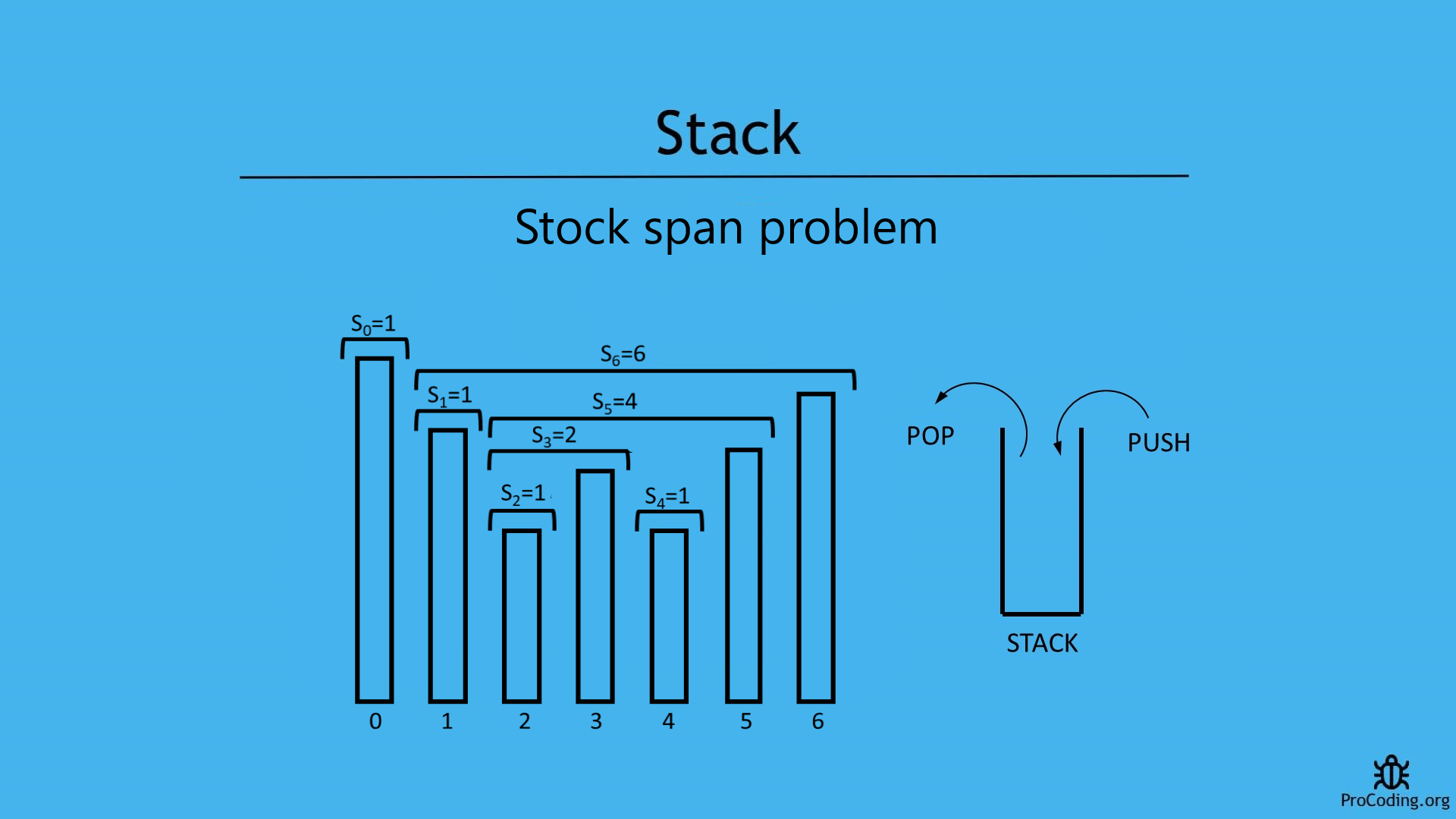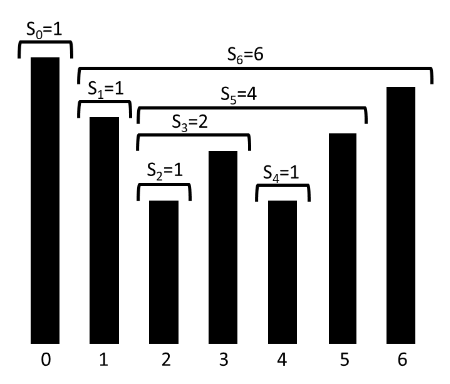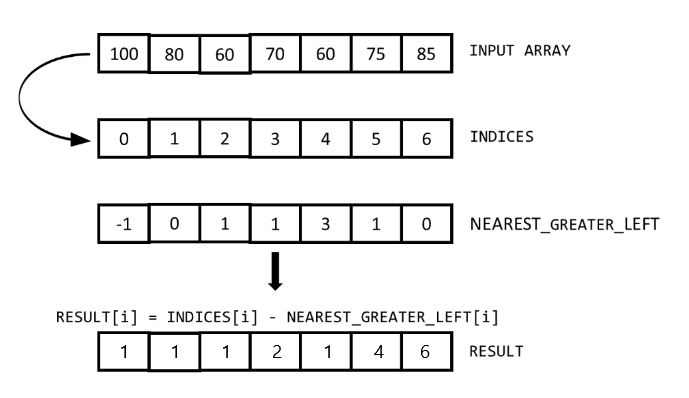# Stock span problem

Abhishek Kumar Category: Data StructuresTags: datastructures stack

Stock span problem is a financial problem mostly asked in interviews and its optimized solution can be obtained using stack.

Here is the solution of stock span problem with explanation using stack and brute force approach.#### Problem

The stock span problem is a type of financial problem based on stocks where daily price of a stock is given.

The span of the stock's price today is defined as the maximum number of consecutive days (starting from today and going backwards) for which the price of the stock was less than or equal to today's price.##### Example -
```Input: [100, 80, 60, 70, 60, 75, 85]
Output: [1, 1, 1, 2, 1, 4, 6]

Input: [31, 27, 14, 21, 30, 22]
Output: [1, 1, 1, 2, 4, 1]```

We will see two ways to solve the problems

#### Brute-force approach (using nested loops)

In this approach we will use two loops. Outer loop will iterate over all the array items and inner loop will iterate over all the previous elements of currently pointed by outer loop. Inner loop will check, if any greater element will be found then loop will be terminated.

``````def stock_span(arr):
result = []
for i in range(len(arr)):
flag = True
count = 0
for j in range(i, -1, -1):
if arr[j] <= arr[i]:
count += 1
else:
flag = False
break

if not flag or j <= 0:
result.append(count)

return result

print(stock_span([100, 80, 60, 70, 60, 75, 85]))``````
``````function stockSpan(arr) {
const result = [];
for (var i = 0; i < arr.length; i++) {
var flag = true;
var count = 0;
for (var j = i; j >= 0; j--) {
if (arr[j] <= arr[i]) {
count++;
}
else {
flag = false;
break;
}
}
if (!flag || j <= 0)
result.push(count);
}
return result;
}

console.log(stockSpan([100, 80, 60, 70, 60, 75, 85]));``````

#### Output

Output

`[1, 1, 1, 2, 1, 4, 6]`

Although, we have solved this problem using loops but this is not an optimized solution.
Because time complexity of this solution is O(n2).

#### Using stack

You can observe that this problem is very much similar to Nearest greater element to left because in this problem we are trying to find the nearest greater element to left.

But instead of the greater element, we are storing the index of greater element and then we will subtract index of greater from the index of current element.##### Program
``````class Stack:
def __init__(self):
self.stack = []

def isEmpty(self):
return len(self.stack) == 0

def push(self, num):
self.stack.append(num)

def pop(self):
if self.isEmpty():
raise Exception('Stack Underflow')
return self.stack.pop()

def peek(self):
if self.isEmpty():
return None
return self.stack[-1]

def stock_span(arr):
stack = Stack()
result = []

for i in range(0, len(arr)):
if stack.isEmpty():
result.append(-1)
stack.push([arr[i], i])
elif not stack.isEmpty():
while not stack.isEmpty() and arr[i] > stack.peek():
stack.pop()
if stack.isEmpty():
result.append(-1)
else:
result.append(stack.peek())
stack.push([arr[i], i])

output = []
for index, elem in enumerate(result):
output.append(index - elem)

return output

arr = [100, 80, 60, 70, 60, 75, 85]
print(stock_span(arr))``````
``````class Stack {
constructor() {
this.stack = [];
}

isEmpty() {
return this.stack.length === 0;
}

push(num) {
this.stack.push(num);
}

pop() {
if (this.isEmpty())
throw 'Stack underflow';
return this.stack.pop();
}

peek() {
if (this.isEmpty())
return null;
return this.stack[this.stack.length - 1];
}
}

function stockSpan(arr) {
const stack = new Stack();
const result = [];

for (let i = 0; i < arr.length; i++) {
if (stack.isEmpty()) {
result.push(-1);
stack.push([arr[i], i])
} else if (!stack.isEmpty()) {
while (!stack.isEmpty() && arr[i] > stack.peek()) {
stack.pop();
}
if (stack.isEmpty())
result.push(-1);
else
result.push(stack.peek());
stack.push([arr[i], [i]]);
}
}

const output = [];
for (let i = 0; i < result.length; i++)
output.push(i - result[i]);

return output;
}

const arr = [100, 80, 60, 70, 60, 75, 85];
console.log(stockSpan(arr));``````

#### Output

Output

`[1, 1, 1, 2, 1, 4, 6]`

AUTHOR### Abhishek Kumar

Software engineer | Blogger | Keen learner
Python | Django | JavaScript | React | Next.js | Express.js | C
Passionate about learning and experimenting with new things and open to more opportunities and collaborations.